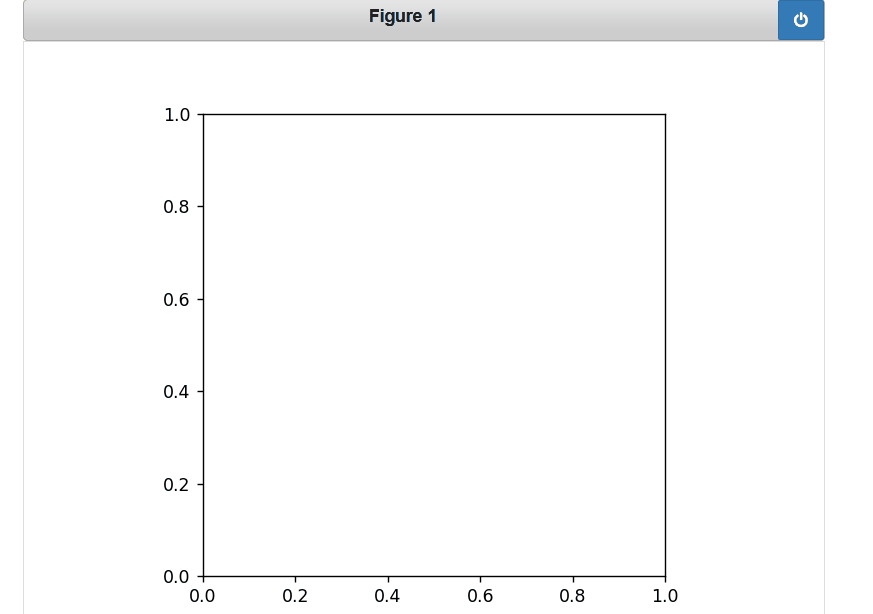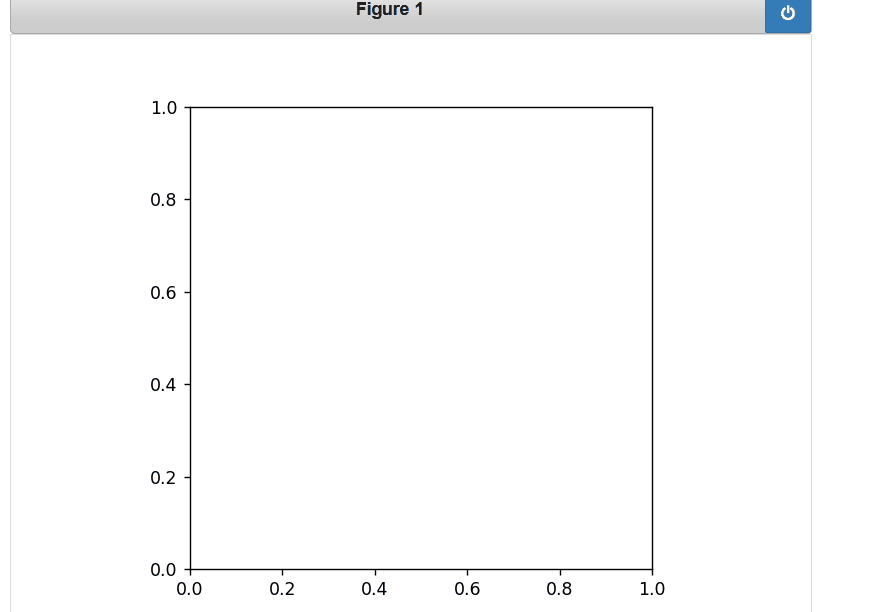Open in App
Not now

# Event Handling in Matplotlib

• Last Updated : 18 Oct, 2021

Event based System usually is part of a recurring set of patterns. Often, they comprise of the following :

• An incoming event
• A mechanism that is used to respond to an event
• A looping construct (e.g. while loop, listener, and the message dispatch mechanism)

The events that are triggered are also a bit richer, including information like which Axes the event occurred in. The events also understand the Matplotlib coordinate system and report event locations in both pixel and data coordinates.

Syntax:

figure.canvas.mpl_connect( Event_name , callback function or method)

Parameters:

• Event_name: It could be any from the below table
• callback_function: It will define to handle the event.

## List of events

Note: That the classes are defined in matplotlib.backend_bases

## MOUSE EVENT

• button_press_event: This event involves a mouse button press
• button_release_event: This event involves a mouse button release
• scroll_event: This event involves scrolling of the mouse
• motion_notify_event: This event involves a notification pertaining to the mouse movement

Example:

We have used the mpl_connect method, which must be called if you want to provide custom user interaction features along with your plots. This method will take two arguments:

• A string value for the event, which can be any of the values listed in the Event Name column of the preceding table
• A callback function or method

## Python3

 `# importing the necessary modules``from` `IPython.display ``import` `Image``import` `matplotlib.pyplot as plt``import` `matplotlib as mpl``import` `numpy as np``import` `time``import` `sys``import` `random``import` `matplotlib``matplotlib.use(``'nbagg'``)`` ` ` ` `class` `MouseEvent:``   ` `    ``# initialization``    ``def` `__init__(``self``):``        ``(figure, axes) ``=` `plt.subplots()``        ``axes.set_aspect(``1``)``        ``figure.canvas.mpl_connect(``'button_press_event'``, ``self``.press)``        ``figure.canvas.mpl_connect(``'button_release_event'``, ``self``.release)`` ` `    ``# start event to show the plot``    ``def` `start(``self``):``        ``plt.show()  ``# display the plot`` ` `    ``# press event will keep the starting time when u ``    ``# press mouse button``    ``def` `press(``self``, event):``        ``self``.start_time ``=` `time.time()`` ` `    ``# release event will keep the track when you release``    ``# mouse button``    ``def` `release(``self``, event):``        ``self``.end_time ``=` `time.time()``        ``self``.draw_click(event)`` ` `    ``# drawing the plot``    ``def` `draw_click(``self``, event):``        ``# size = square (4 * duration of the time button ``        ``# is keep pressed )``        ``size ``=` `4` `*` `(``self``.end_time ``-` `self``.start_time) ``*``*` `2``         ` `        ``# create a point of size=0.002 where mouse button ``        ``# clicked on the plot``        ``c1 ``=` `plt.Circle([event.xdata, event.ydata], ``0.002``,)``         ` `        ``# create a circle of radius 0.02*size``        ``c2 ``=` `plt.Circle([event.xdata, event.ydata], ``0.02` `*` `size, alpha``=``0.2``)``        ``event.canvas.figure.gca().add_artist(c1)``        ``event.canvas.figure.gca().add_artist(c2)``        ``event.canvas.figure.show()`` ` ` ` `cbs ``=` `MouseEvent()`` ` `# start the event``cbs.start()`

Output :Example 2:

We are going to add color using the draw_click  method

## Python3

 `def` `draw_click(``self``, event):``   ` `    ``# you can specified your own color list``    ``col ``=` `[``'magneta'``, ``'lavender'``, ``'salmon'``, ``'yellow'``, ``'orange'``]``    ``cn ``=` `random.randint(``0``, ``5``)``     ` `    ``# size = square (4 * duration of the time button ``    ``# is keep pressed )``    ``size ``=` `4` `*` `(``self``.end_time ``-` `self``.start_time) ``*``*` `2``     ` `    ``# create a point of size=0.002 where mouse button ``    ``# clicked on the plot``    ``c1 ``=` `plt.Circle([event.xdata, event.ydata], ``0.002``,)``     ` `    ``# create a circle of radius 0.02*size``    ``c2 ``=` `plt.Circle([event.xdata, event.ydata], ``0.02` `*``                    ``size, alpha``=``0.2``, color``=``col[cn])``    ``event.canvas.figure.gca().add_artist(c1)``    ``event.canvas.figure.gca().add_artist(c2)``    ``event.canvas.figure.show()`

Output:My Personal Notes arrow_drop_up